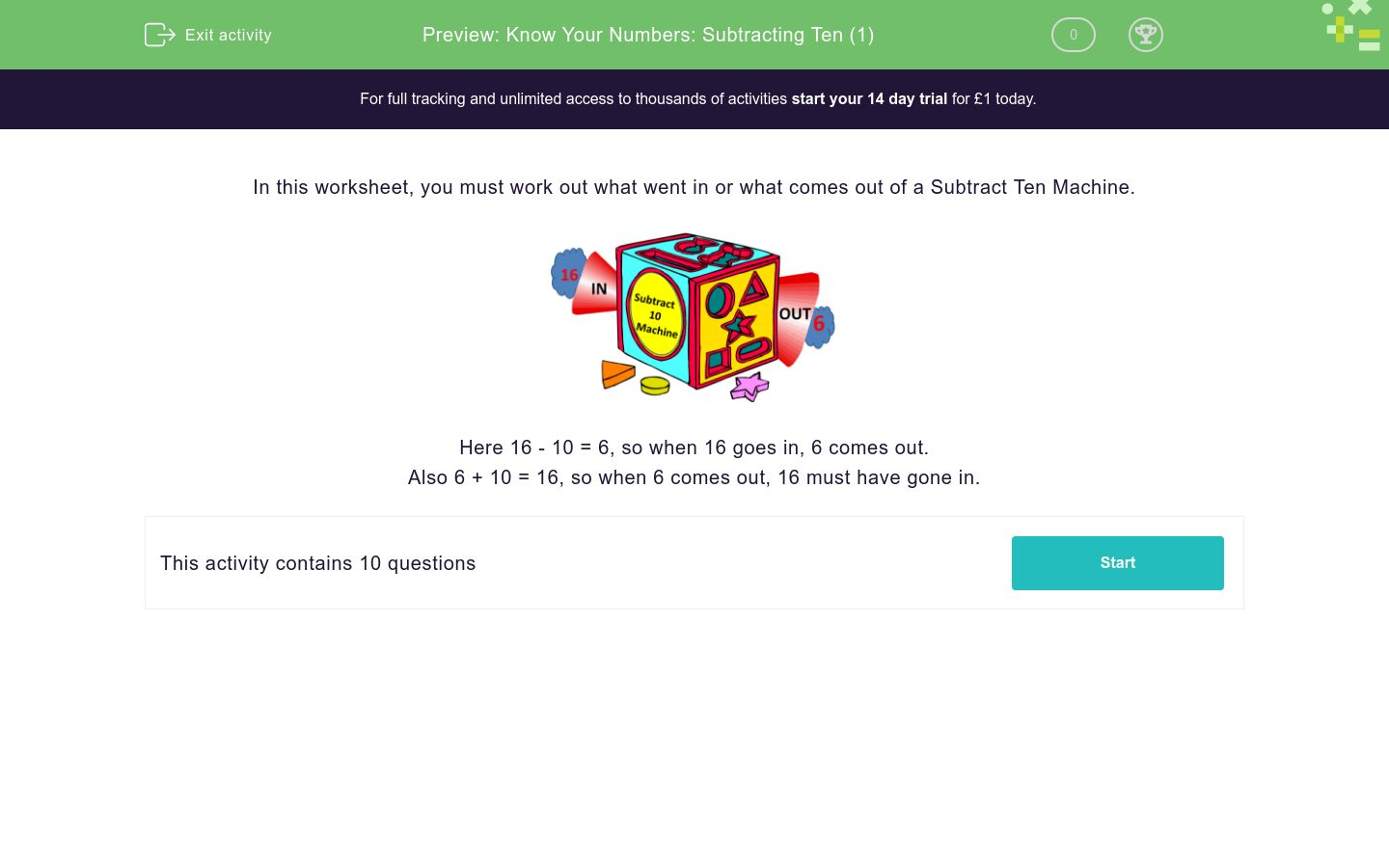# Know Your Numbers: Subtracting Ten (1)

In this worksheet, students work out what comes out of or goes in to a Subtract Ten Machine.Key stage:  KS 1

Curriculum topic:   Number: Addition and Subtraction

Curriculum subtopic:   Solve Add/Subtract Problems to 20

Difficulty level:### QUESTION 1 of 10

In this worksheet, you must work out what went in or what comes out of a Subtract Ten Machine.Here 16 - 10 = 6, so when 16 goes in, 6 comes out.

Also 6 + 10 = 16, so when 6 comes out, 16 must have gone in.

---- OR ----